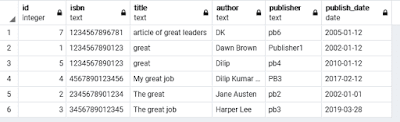### SQL Server- How to get last access/update time for tables in Database?

One day I got a requirement to find last updates of tables in the database. Means I want to get all the tables’ last update in the database and did surfing on google and found the best way. Link:

I am taking reference from the above link and try to explain. See the following query it may help you to find the exact result.

 SELECT OBJECT_NAME(OBJECT_ID) AS DatabaseName, last_user_update,* FROM sys.dm_db_index_usage_stats WHERE database_id = DB_ID( '')

If you want to see the last update of particular the table then use the following query.

 SELECT OBJECT_NAME(OBJECT_ID) AS DatabaseName, last_user_update,* FROM sys.dm_db_index_usage_stats WHERE database_id = DB_ID( '') AND OBJECT_ID=OBJECT_ID('table_name')

### SQL Server - How can we truncate all tables in Database?

sp_msforeachtable can be used to truncate tables from the database. sp_msforeachtable is undocumented means this is not documented by Microsoft it can be changed or modified at any time. It contains two parameters @command1 and @whereand using this procedure we can truncate tables.

If you want to truncate all the tables in the database then use the following query.

 Exec Sp_msforeachtable @command1='Truncate Table ?'

If you want to truncate all the tables of particular schema then use the following query

 Exec Sp_msforeachtable @command1='Truncate Table ?',@whereand='and Schema_Id=Schema_id(''schema_name'')'

### SQL Server - How to find all empty/have values tables

Many times our managers ask to find empty tables and have value tables report. It is time taken to find the tables manually. I am writing some queries which may help you to find empty and have a values table.
The following query helps you to find all empty tables in the database.

1 – See the following query

 SELECT IST.TABLE_NAME , MAX(SI.rows ) TOTAL_RECORDS FROM sysindexes SI, INFORMATION_SCHEMA.TABLES IST WHERE IST.TABLE_NAME = OBJECT_NAME(SI.id)       AND IST.TABLE_TYPE = 'BASE TABLE' GROUP BY IST.TABLE_SCHEMA, IST.TABLE_NAME HAVING MAX(SI.rows)<=0

2- If you want to find all empty tables of particular “schema” then use the following query.

 SELECT IST.TABLE_NAME , MAX(SI.rows ) TOTAL_RECORDS FROM sysindexes SI, INFORMATION_SCHEMA.TABLES IST WHERE IST.TABLE_NAME = OBJECT_NAME(SI.id)       AND IST.TABLE_TYPE = 'BASE TABLE'       AND IST.TABLE_SCHEMA='Schema_Name' GROUP BY IST.TABLE_SCHEMA, IST.TABLE_NAME HAVING MAX(SI.rows)<=0

If you want to find those all tables that have values, then use the following queries.
1 – Query to find all tables that have values.

 SELECT IST.TABLE_NAME , MAX(SI.rows ) TOTAL_RECORDS FROM sysindexes SI, INFORMATION_SCHEMA.TABLES IST WHERE IST.TABLE_NAME = OBJECT_NAME(SI.id)       AND IST.TABLE_TYPE = 'BASE TABLE' GROUP BY IST.TABLE_SCHEMA, IST.TABLE_NAME HAVING MAX(SI.rows)>0

2- If you want to find all tables that have values of particular “schema,” then use the following query.

 SELECT IST.TABLE_NAME , MAX(SI.rows ) TOTAL_RECORDS FROM sysindexes SI, INFORMATION_SCHEMA.TABLES IST WHERE IST.TABLE_NAME = OBJECT_NAME(SI.id)       AND IST.TABLE_TYPE = 'BASE TABLE'       AND IST.TABLE_SCHEMA = 'SCHEMA_NAME' GROUP BY IST.TABLE_SCHEMA, IST.TABLE_NAME HAVING MAX(SI.rows)>0

 ;WITH TBL AS (    SELECT       SUM(row_count) AS ROW_COUNT,       OBJECT_NAME(OBJECT_ID) AS TABLE_NAME,       OBJECT_SCHEMA_NAME(OBJECT_ID) AS TABLE_SCHEMA    FROM       sys.dm_db_partition_stats    WHERE       INDEX_ID = 0 OR INDEX_ID= 1              GROUP BY       OBJECT_ID ) SELECT * FROM TBL WHERE ROW_COUNT = 0

You can filter for schema as below.

 ;WITH TBL AS (    SELECT       SUM(row_count) AS ROW_COUNT,       OBJECT_NAME(OBJECT_ID) AS TABLE_NAME,       OBJECT_SCHEMA_NAME(OBJECT_ID) AS TABLE_SCHEMA    FROM       sys.dm_db_partition_stats    WHERE       INDEX_ID = 0 OR INDEX_ID= 1              GROUP BY       OBJECT_ID ) SELECT * FROM TBL WHERE ROW_COUNT = 0 AND TABLE_SCHEMA='Schema_Name'

If you wish to find all tables which have values so please change in above query ROW_COUNT >0

### How to copy variable from one package to another SSIS package

We can easily copy and paste task, transformations, connection manager from one package to another, but like this, you cannot copy and paste variables from one to another package. If you have to use the same variable for another package it may take time to type variable’s name one by one.

One solution for this I am described below, we can copy and paste the variable from one package to another Package. Suppose we have a package pck1 and we need to copy all variable from package pck1 and paste to package pck2.

To copy the above variable, we have to open the XML code of pck1. How can we open the package in code mode? Please see the following image.

Copy the variable code shown as below.

Same as open package pck2 in code mode and replace variables attribute with copied code as below.

After replacing code and save, the package will look like as below.

### PostgreSQL-Query: Sort result set by specific field values using ORDER BY Clause

Problem: Suppose we have a book_inventory table which has some columns such as id, isbn, title, author, publisher, publish_date, etc.. whe...